# vs.eyeandcontacts.com

## Chapter 7 Cubes and Cube Roots Exercise 7.1

Question 1: Which of the following numbers are not perfect cubes?
(i) 216
(ii) 128
(iii) 1000
(iv) 100
(v) 46656

(i)Therefore, 216 is a perfect cube.

(ii)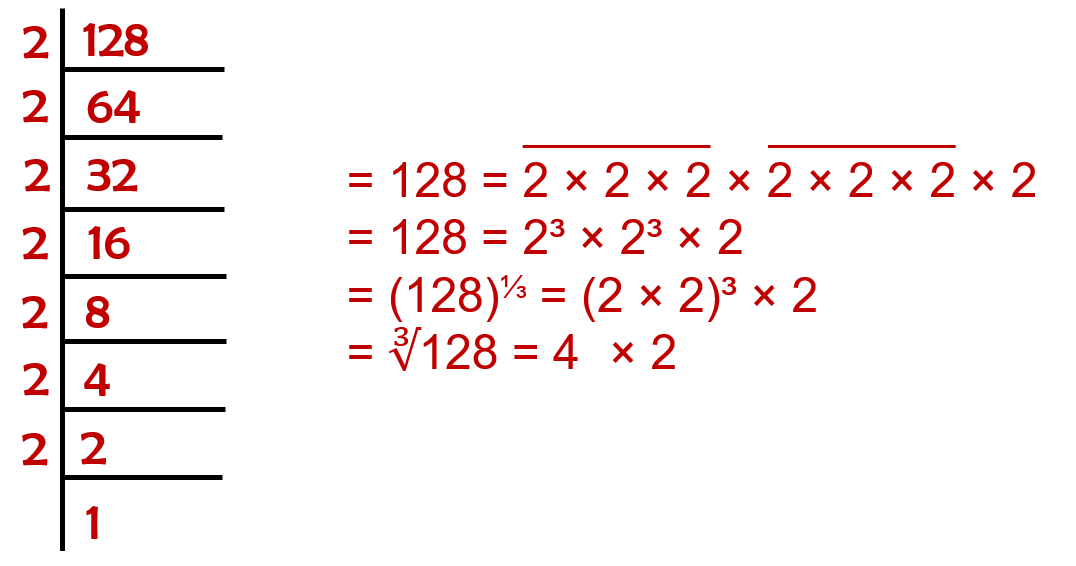Therefore, 128 is not a perfect square.

(iii)Therefore, 1000 is a perfect square.

(iv)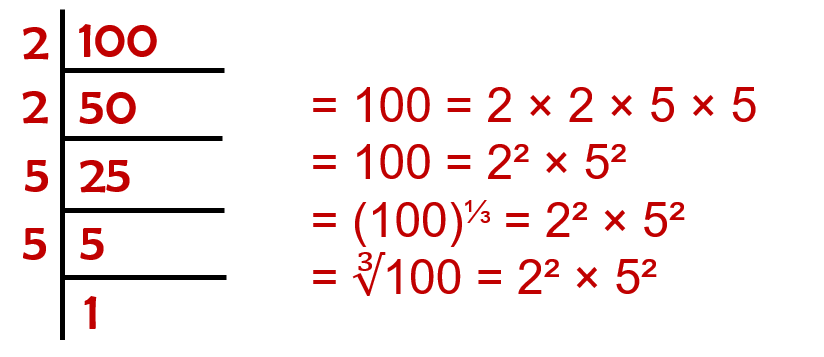Therefore, 100 is not a perfect square.

(v)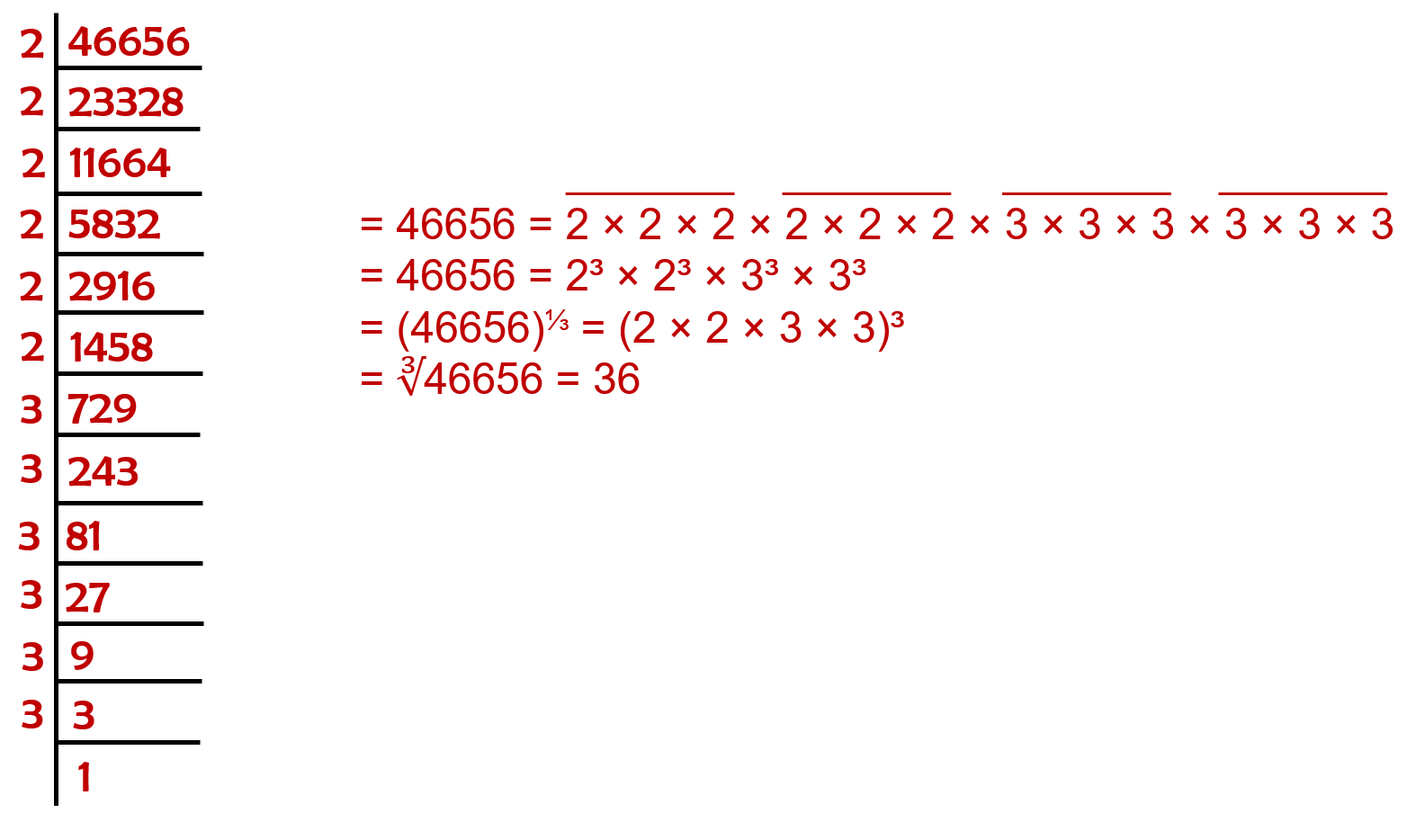Therefore, 46656 is a perfect square.

Question 2: Find the smallest number by which each of the following numbers must be multiplied to obtain a perfect cube.
(i) 243
(ii) 256
(iii) 72
(iv) 675
(v) 100
(i)Therefore, the smallest number by which 243 must be multiplied to obtain a perfect cube is 3.

(ii)Therefore, the smallest number by which 256 must be multiplied to obtain a perfect cube is 2.

(iii)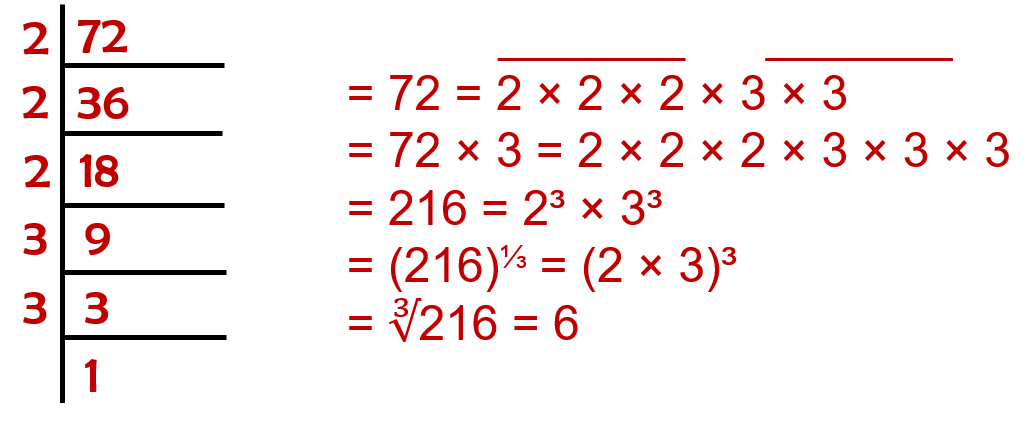Therefore, the smallest number by which 72 must be multiplied to obtain a perfect cube is 3.

(iv)Therefore, the smallest number by which 675 must be multiplied to obtain a perfect cube is 5.

(v)Therefore, the smallest number by which 100 must be multiplied to obtain a perfect cube is 10.

Question 3: Find the smallest number by which each of the following numbers must be divided to obtain a perfect cube.
(i) 81
(ii) 128
(iii) 135
(iv) 192
(v) 704

(i)Therefore, the smallest number by which 81 must be divided to obtain a perfect cube is 3.

(ii)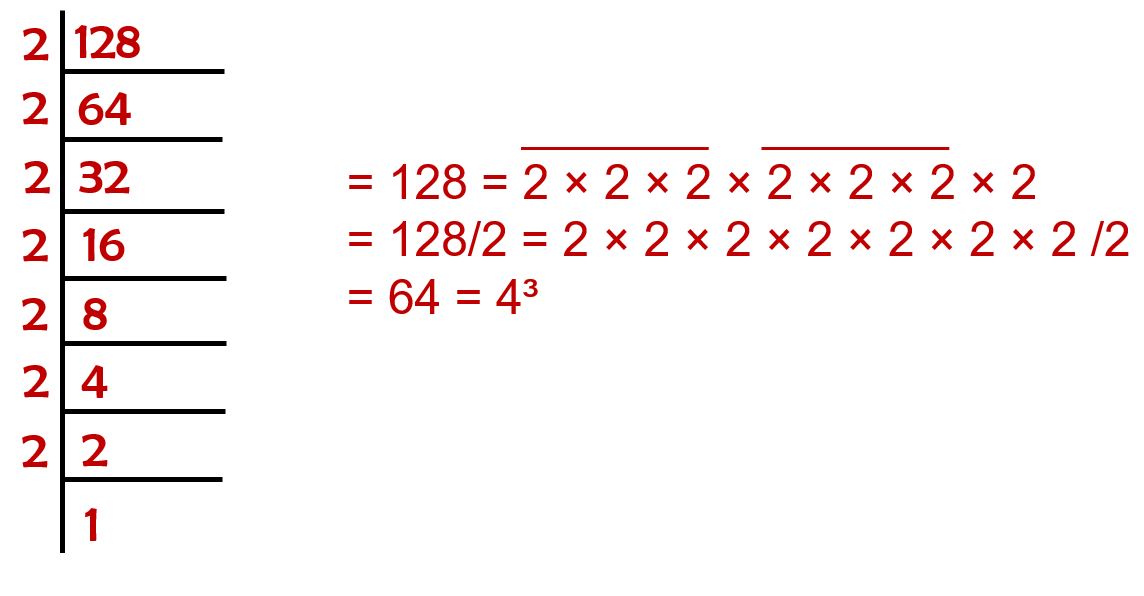Therefore, the smallest number by which 128 must be divided to obtain a perfect cube is 2.

(iii)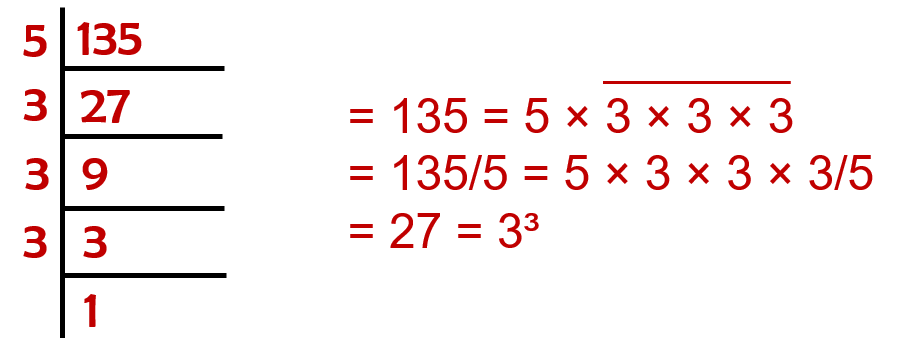Therefore, the smallest number by which 135 must be divided to obtain a perfect cube is 5.

(iv)Therefore, the smallest number by which 192 must be divided to obtain a perfect cube is 3.

(v)Therefore, the smallest number by which 704 must be divided to obtain a perfect cube is 11.

Question 4: Parikshit makes a cuboid of plasticine of sides 5 cm, 2 cm, 5 cm. How many such cuboids will he need to form a cube?

Volume of cuboid × number of cuboids = volume of cube
lbh × number of cuboids = (edge)³
5 × 2 × 5 × (x) = (edge)³
5 × 2 × 5 × (5 × 2 × 2) = (edge)³
5 × 2 × 5 × 20 cuboids = 5³ × 2³

Therefore, Parikshit require 20 cuboids to make a cube.